Type
Solution Manual
Book Title
Statics and Mechanics of Materials 2nd Edition
ISBN 13
978-0073398167

### 978-0073398167 Chapter 8 Solution Manual Part 3

February 21, 2020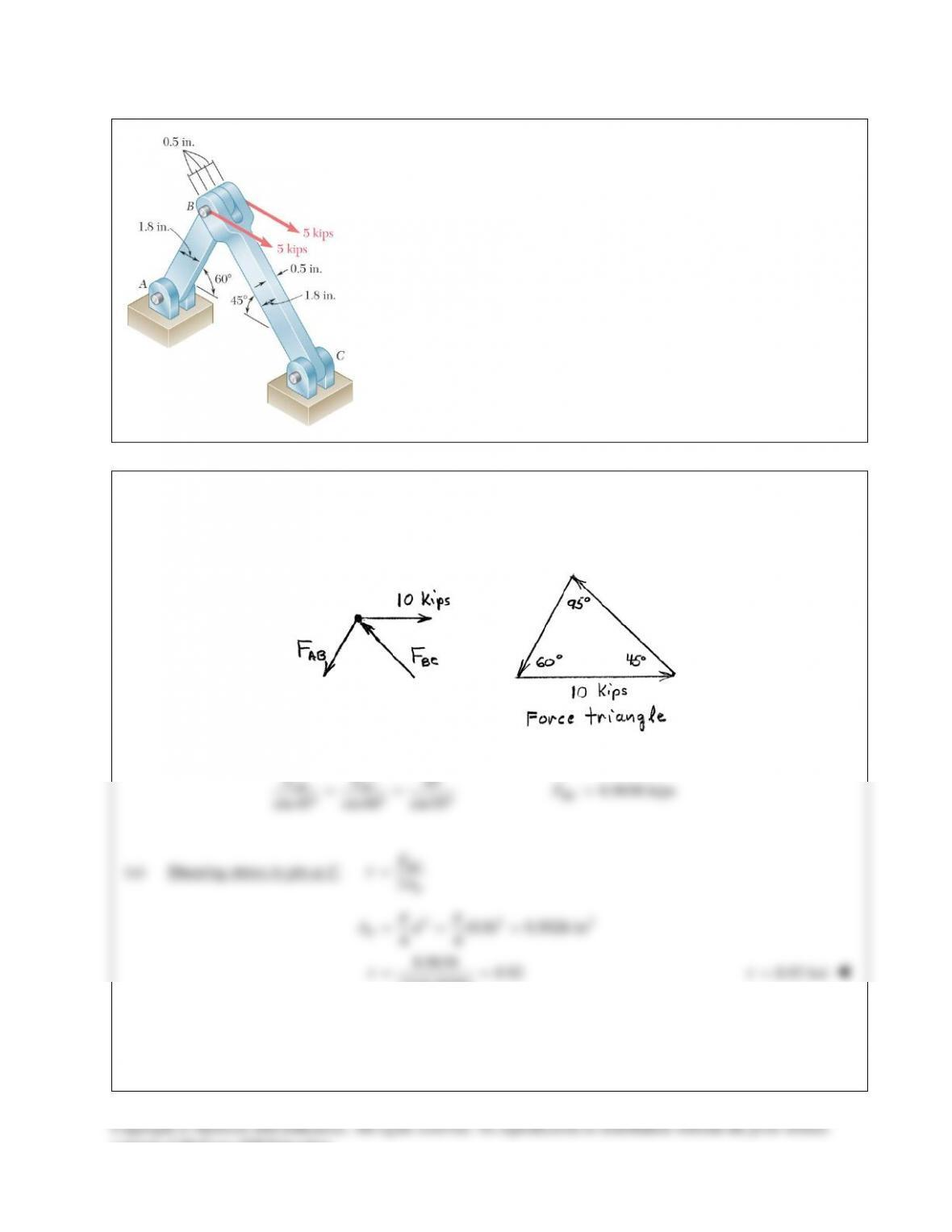PROBLEM 8.21
For the assembly and loading of Prob. 8.8, determine (a) the average
shearing stress in the pin at C, (b) the average bearing stress at C in
member BC, (c) the average bearing stress at B in member BC.
PROBLEM 8.8 Two horizontal 5-kip forces are applied to pin B of the
assembly shown. Knowing that a pin of 0.8-in. diameter is used at each
connection, determine the maximum value of the average normal stressSOLUTION Continued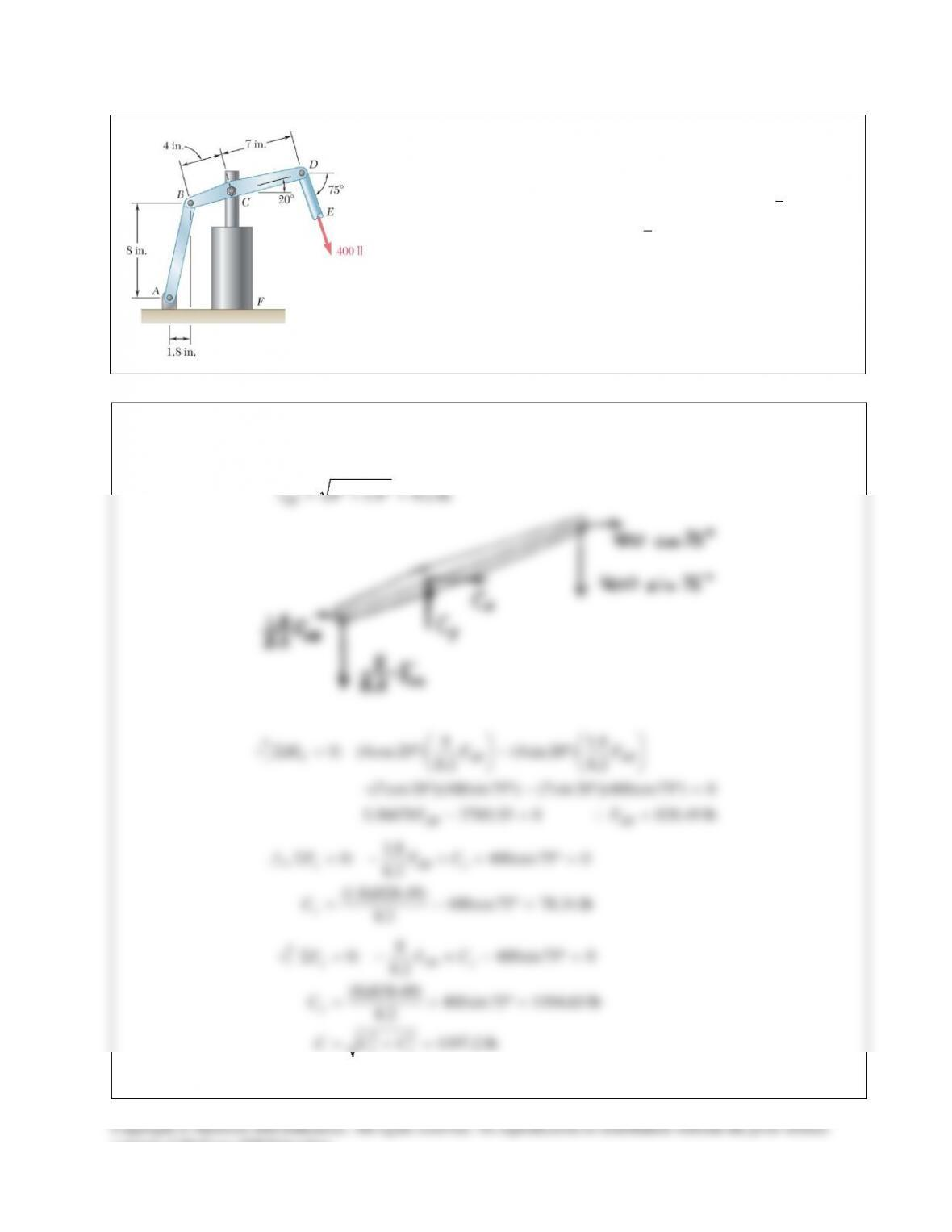PROBLEM 8.22
The hydraulic cylinder CF, which partially controls the position of rod
DE, has been locked in the position shown. Member BD is
5
8
in. thick
and is connected to the vertical rod by a
3
8
-in.-diameter bolt. Determine
(a) the average shearing stress in the bolt, (b) the bearing stress at C in
member BD.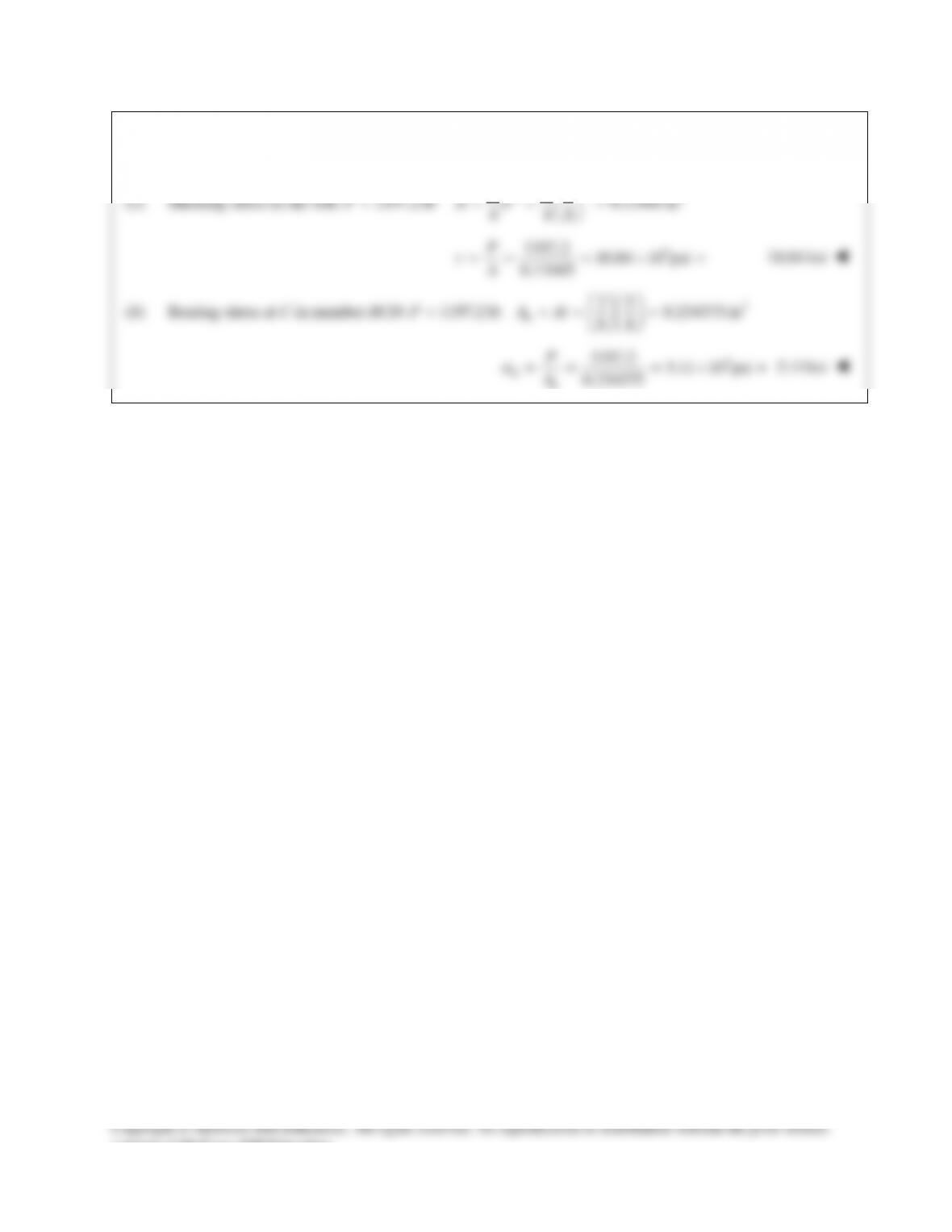SOLUTION Continued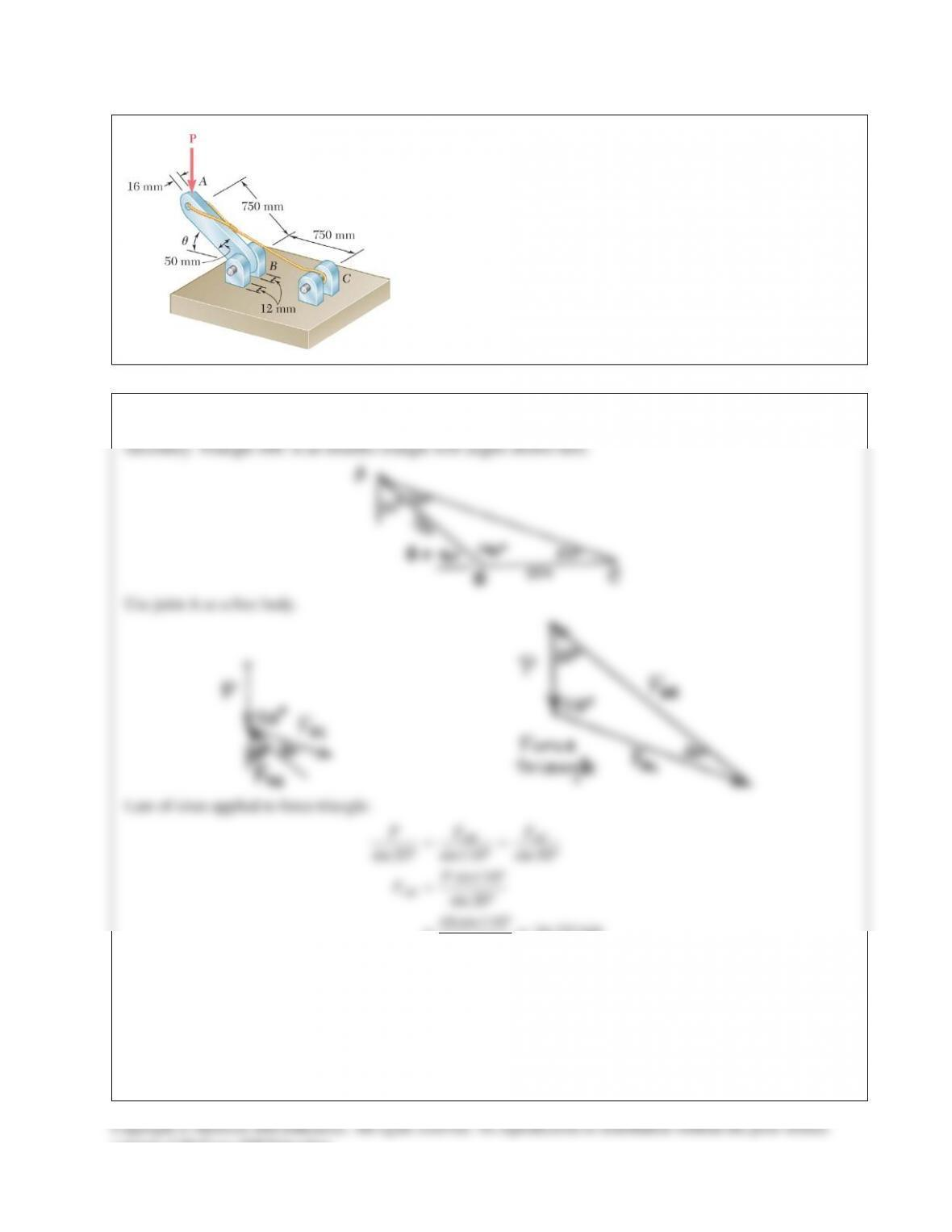PROBLEM 8.23
Knowing that
θ
= 40° and P = 9 kN, determine (a) the smallest
allowable diameter of the pin at B if the average shearing stress in
the pin is not to exceed 120 MPa, (b) the corresponding average
bearing stress in member AB at B, (c) the corresponding average
bearing stress in each of the support brackets at B.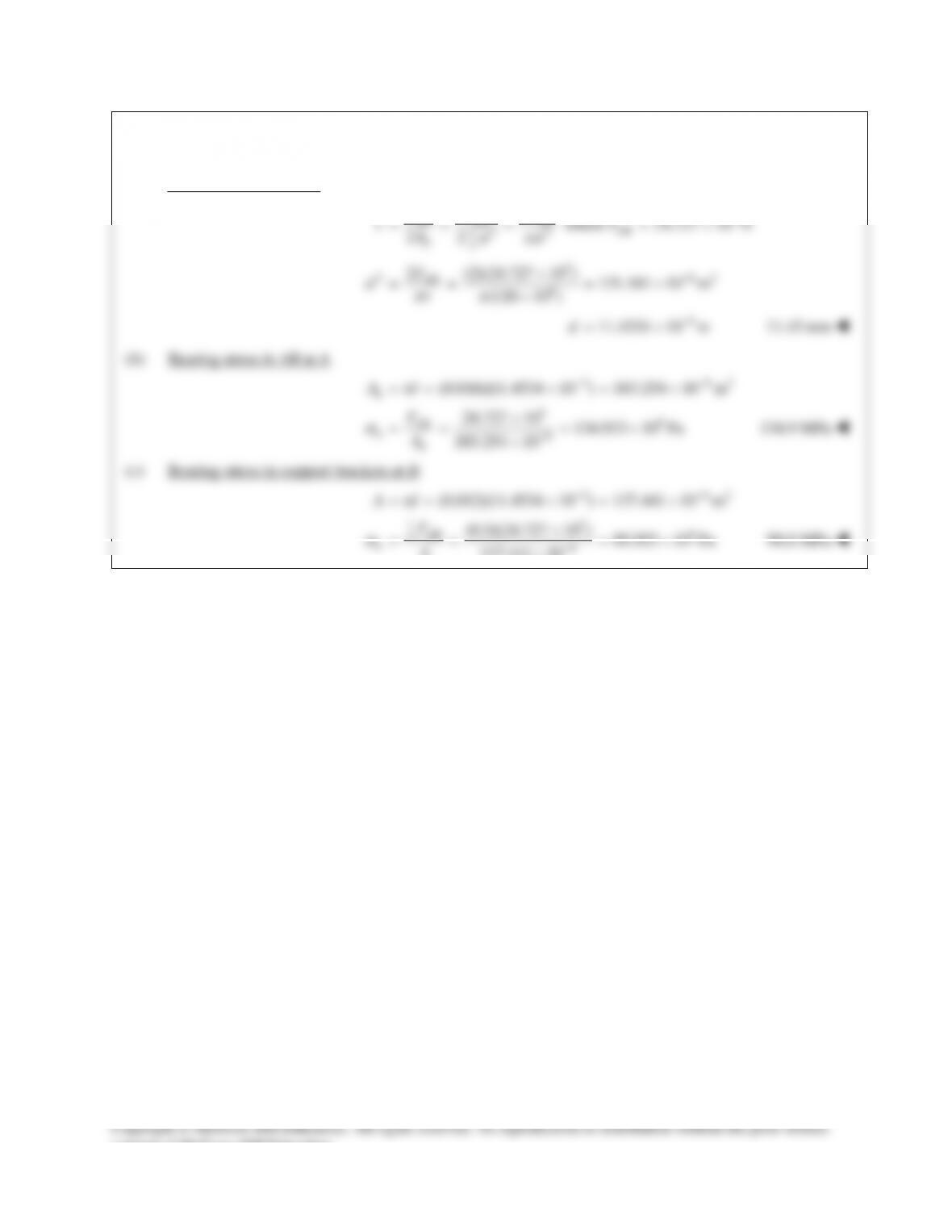SOLUTION Continued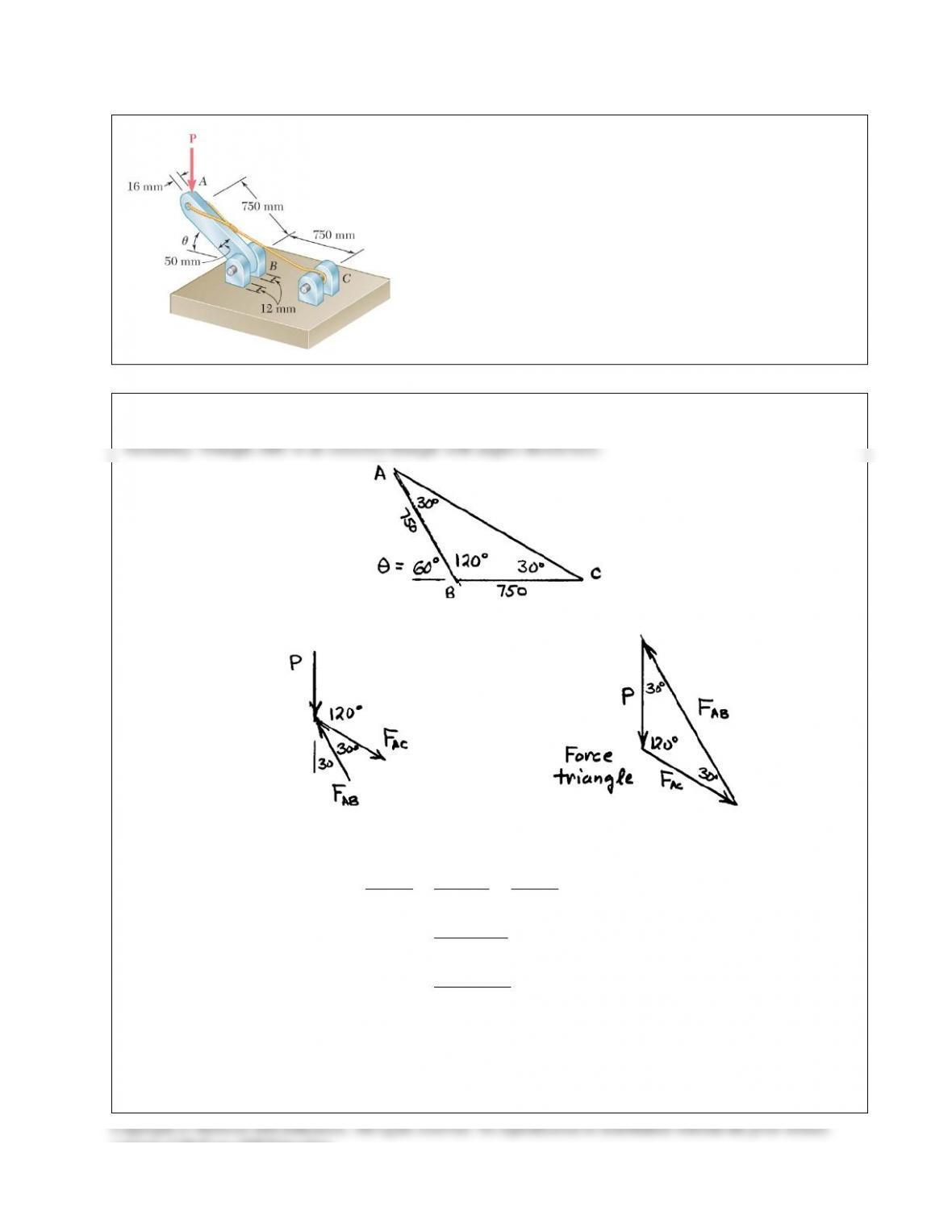PROBLEM 8.24
Determine the largest load P which may be applied at A when
θ
= 60°, knowing that the average shearing stress in the 10-mm-
diameter pin at B must not exceed 120 MPa and that the average
bearing stress in member AB and in the bracket at B must not
exceed 90 MPa.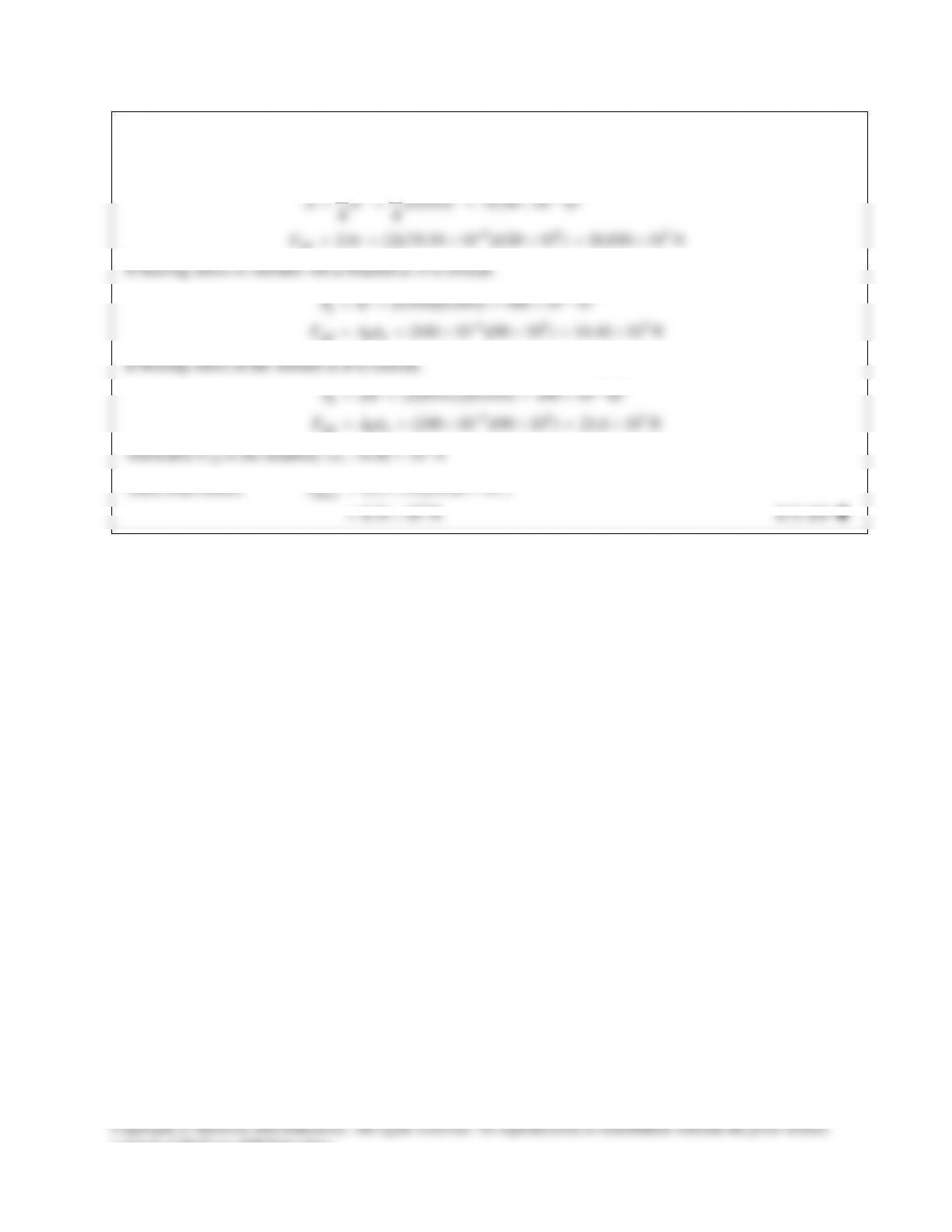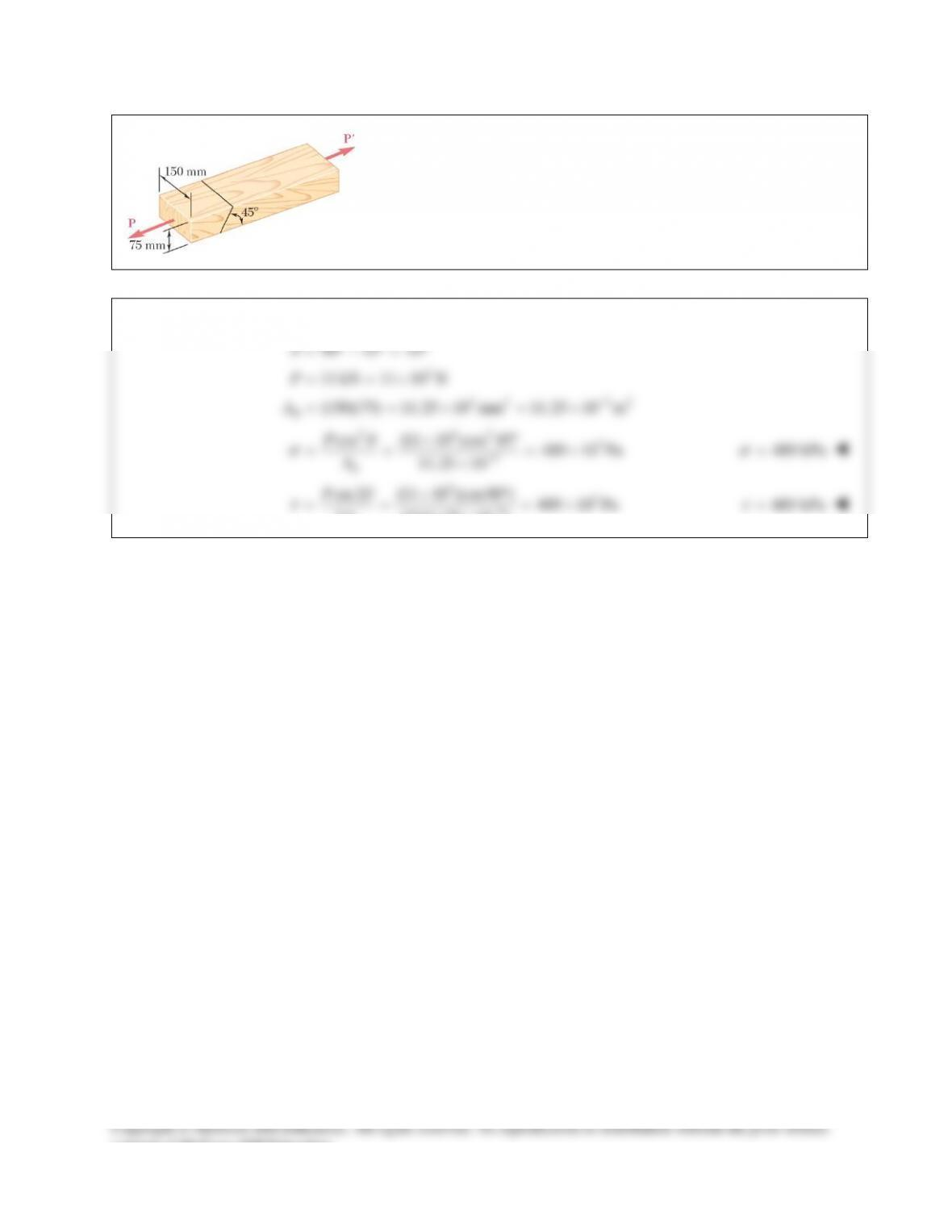PROBLEM 8.25
Two wooden members of uniform rectangular cross section are joined
by the simple glued scarf splice shown. Knowing that P = 11 kN,
determine the normal and shearing stresses in the glued splice.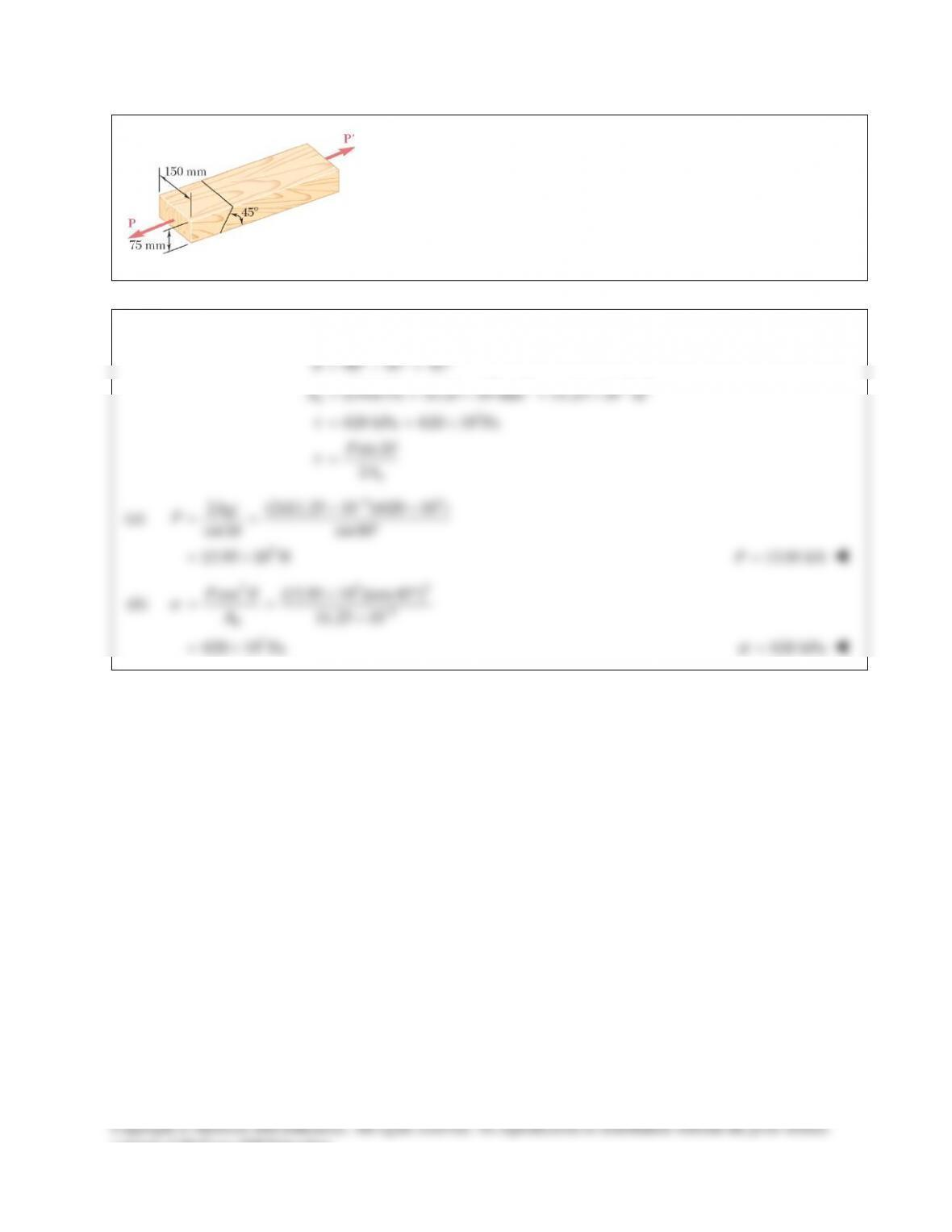PROBLEM 8.26
Two wooden members of uniform rectangular cross section are joined
by the simple glued scarf splice shown. Knowing that the maximum
allowable shearing stress in the glued splice is 620 kPa, determine
(a) the largest load P that can be safely applied, (b) the corresponding
tensile stress in the splice.